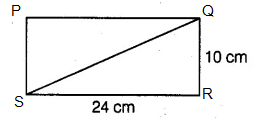# Length of one of the diagonals

Question:

Length of one of the diagonals of a rectangle whose sides are 10 cm and 24 cm is

(a) 25 cm

(b) 20 cm

(c) 26 cm

(d) 3.5 cm

Solution:

(c) 26 cmPQRS is a rectangle,

Where SR = 24 cm, QR = 10 cm

Now, consider the triangle QRS

From the rule of Pythagoras theorem,

QS2 = SR2 + QR2

QS2 = 242 + 102

QS2 = 576 + 100

QS2 = 676

QS = √676

QS = 26 cm